# MCAT Physical : Combining Lenses

## Example Questions

### Example Question #10 : Mirrors And Lenses

In a microscope has a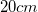tube length and objective lens with a focal length of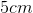. The viewer's eye is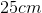from the objective lens, and they desire a magnification of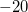. What must the focal length of the eyepiece lens be to achieve this magnification?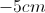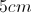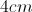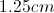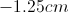Explanation:

The total magnification of a compound microscope is the product of the objective lens magnification and the eyepiece magnification.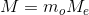Objective magnification and eyepiece magnification are given by the following equations: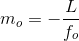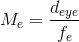We are given the tube length and focal length of the objective lens, allowing us to solve for its magnification.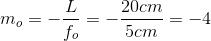We also know the distance of the viewer's eye. Use this value in the eyepiece magnification equation.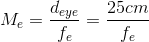Finally, combine the eyepiece magnification and objective lens magnification into the original equation for total magnification.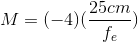Use the given value for total magnification to solve for the focal length of the eyepiece lens.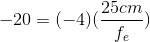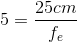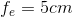### Example Question #1 : Combining Lenses

A compound microscope consists of an eyepiece with an angular magnification of 25 and an objective lens of unknown focal length. If the length of the microscope tube is 25cm, what magnitude of objective focal length is necessary to achieve an overall magnification of 500?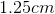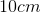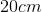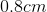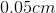Explanation:

Relevant equations:= length of microscope tube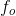= focal length of objective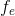= focal length of eyepiece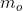= linear magnification of objective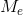= angular magnification of eyepiece= total magnification of microscope

Given: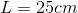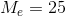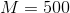Step 1: Plug the expression forinto the equation for total magnification,.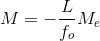Step 2: Rearrange to isolate the unknown,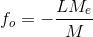Step 3: Plug in given quantities, taking the absolute value to find the magnitude of.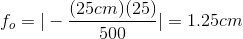### Example Question #11 : Mirrors And Lenses

What diameter of telescope objective lens is necessary to resolve two stars that primarily emit 600nm light and have an angular separation of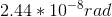?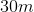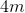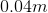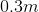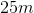Explanation:

Relevant equations: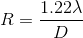= angular separation of sources, in radians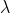= wavelength of light emitted by sources, in meters= diameter of telescope

Step 1: Rearrange equation to isolate the unknown,: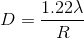Step 2: Plug in the given numbers for wavelength and angular separation: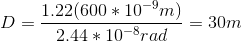### All MCAT Physical Resources